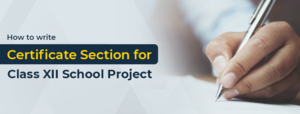SSC CGL 2020 Exam Topic - Quantitive Abilities - Study24x7Default error msg

New to Study24x7 ?

# SSC CGL 2020 Exam Topic - Quantitive Abilities

Updated on 15 February 2020SSC Preparation Strategies & S
Updated on 15 February 2020

## Quantitative Abilities

The SSC has notified SSC CGL new vacancy 2020 through its website.

The candidates need to practice hard to score well in the exam.

## Regular Polygons

• Regular polygons are the geometrical structures where all the sides and angles of the structure are equal.
• It includes triangle (Regular polygon with 3 sides), quadrilateral (Regular Polygon with 4 sides), Pentagons (Regular Polygon with 5 sides), etc.
• These are also called as an n-sided polygon, where n is the number of sides in a polygon.

For example, a triangle is a 3- sided polygon with n = 3.

• There may be to types of regular polygons and these two are star and convex.

## Basic properties of Regular Polygons are as follows:

1. Symmetry: These types of regular polygons have a symmetrical reflection.
2. The sum of each interior and exterior angle at a single vertex is always 1800.
3. The exterior angle of regular polygon must be 3600/n, where n is the side of a regular polygon.
4. Number of diagonals in a regular polygon can be calculated from the formula 12*n* (n-3) where n is the side of a regular polygon. For example,

In a square where n=4,

The number of diagonals are = 12* 4* (4-3)

= 12*4*1

= 2

## Right Prism (SSC CGL 2020 Exam)

• Right Prism is the solid geometrical structure bounded by polygon at its base and parallelograms at the other two sides and sides are perpendicular (900) to the ends.• It is a 3- dimensional structure.
• On the basis of different polygons the name of right prism changes.

For example, when the base polygon is a triangle, it is called the right triangle prism or when the base polygon is rectangular it is called right rectangular prism and so on.

## Formulas related to Right Prism are as follows:

1. Volume of a Right Prism= Area of Base Polygon * Height of the right prism
2. Surface area of a Right Prism= (2 * Area of base Polygon) + surface area of parallelograms

## Right Circular Cone (SSC CGL 2020 Exam)

• The right circular cone is a geometrical structure of a cone that has a circular section and its axis is perpendicular (900) to the base of the cone.
• It is a cone with a circular base.• The right circular cone also has slant height as a measurement denoted by l which joins the vertex of the cone to the exterior point of the circular base of a right circular cone.
• All measured elements in a right circular cone are equal.
• A triangle formed from the vertex to the base of the right circular cone always forms an isosceles triangle.

Formulas related to Right Circular Cone are as follows:

1. Slant height of Right Circular Cone

Slant height l=h2+r2

2. Lateral Surface Area of Right Circular Cone

Lateral Surface Area = πrl

3. Total Surface Area of a Right Circular Cone

Total Surface Area = π (r+ l) r

4. Volume of Right Circular Cone:

Volume = 13πr2h

## All the best to all the aspirants of SSC-CGL2020!

Write a comment...Trending ArticlesBy CBSE CLASS 8By CBSE CLASS 10By Edubabble LLPBy CBSE CLASS 7
Related PostsServicesNeed Some Help!Connect